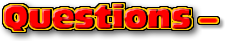ChaptersThis text is meant to accompany class discussions. It is not everything there is to know about the basics of electricity. It is meant as a  prep for class. More detailed notes and examples are given in the class notes, presentations, and demonstrations (click here.)Click for the questions that go with this reading
Vector Math

There two ways to add and subtract vectors, graphically and by components.

Graphically Adding Vectors

An out-of-town driver stops in front of the yellow building and asks for directions to the green building. Taking into account one way streets and closed off streets due to construction, the following directions are given.

 A Drive straight for 1 block; turn left B Drive straight for 2 blocks; turn right C Drive straight for 3 blocks; turn left D Drive straight for 1 block; stop

These directions are a set of vectors. Each step of the directions has a magnitude and direction. When connected they look like the map below. Notice how each vector connects tip to tail.If you had a personal helicopter, then you could fly directly to the ending location. You directions would be fly 5.7 blocks 43 degrees north of pointing due east. Click the "Go" button below to the helicopter in action.

Notice how each set of directions start in the same place and end in the same place. This means they both have the same direction and magnitude. They are equal. Multiple vectors or sets of vectors are equal if they have the same magnitude and direction. In math terms these to sets of directions are written as,In the "typical" algebra class, this is written as "A+B+C+D = R." That is because the typical algebra class only uses scalers to describe quantities. The arrows above there letters mean that these variables are vectors, not scalers. This also implies when describing the answer "R," the answer will have two parts, a magnitude and a direction.( Note: textbooks and other resources will often use a bold face letter instead of arrows of the top of the variables to indicate a vector quantity.)

"R" is the result of adding up the vectors, A, B, C, and D. However, since the answer is a vector,"R" is called the "resultant." The resultant is the vector answer. This means the answer has both magnitude and direction. The answer might look something like "5 m, right," or "3 lbs, up," or "6 m/s, 31 degrees North of West."

The A+B+C+D is the addition operation in math. Notice how the vectors touch each other. They are drawn "tip-to-tail." The answer is found by drawing the resultant from the very beginning to the very end. Try it for yourself below.

Try it again with the same three vectors ...only this time start with different vector and then add the others to it.

Add the vectors a third time. This time start with a different vector. The third vector.

Notice a couple of things about your vector addition.

• The resultant has the same starting and ending points as the vectors placed tip-to-tail.
• It is okay for the resultant to cross other vectors when it is drawn.
• The order of operations does not matter for addition. (This is the commutative property.)